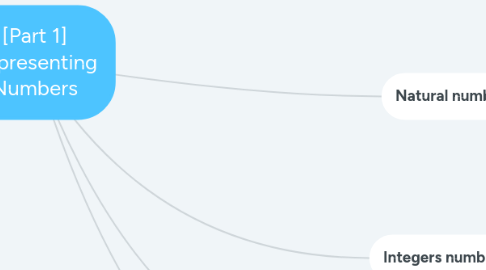# [Part 1] Representing Numbers

Get Started. It's Free[Part 1] Representing Numbers## 1. Integers numbers (Z, Unit 2)

### 1.1. Two's Complement

1.1.1. Read a sequence of bits as an integer

1.1.1.1. Method 1: Convert the sequence in a natural number x, if the first bit is 0 you are done and the result is x, otherwise the number is -(2^n-x) where n is the number of bits

1.1.1.2. Method 2: if the first bit is 0 convert the sequence in a natural number and this is the result, otherwise read the bits from the right, copy them until you find a one, when you find a one copy also the one, but all the other bits should be negated (= 1 becomes 0 and 0 becomes 1), convert the sequence in a natural number x, your result is -x

1.1.2. Find the sequence corresponding to an integer

1.1.2.1. If the number x is non-negative just use the inverse of Horner's algorithm, otherwise write -(2^n - x) = -x', find x' and use the inverse of Horner's algorithm to find the bits of the sequence

## 2. Real numbers (R, Units 4)

### 2.1. Float Format

2.1.1. Read a sequence of bits as a float number

2.1.1.1. The first bit defines the signature, the next 8 bits define the exponent and the remaining 23 the mantissa. If the 8 bits of the exponent are all zeros, the exponent is -126 and the mantissa is 0.[bits of the mantissa] otherwise, the exponent is the horner[bits of the exponent]-127 and the mantissa is 1.[bits of the mantissa]

2.1.2. Find the sequence of bits corresponding to the a given number

2.1.2.1. The sign of the number x tells you about the first bit. Then, forget about the sign of x, and convert it in base 2. This means converting its integer part with the inverse of Horner for natural number (mod) and its fractional part with the inverse of Horner for fractional number. Once you are done with that, you shoul try to write the number in scientific notation with the mantissa normalized to one. Write the exponent as k-127 and represent k as a natural number with 8 bits. These will be the bits of the exponent, while the first 23 bits of the mantissa will be the remaining ones in the sequence (remember that the float format is doint rounding). If this approach leads to a k<=0, your number is too small for the mantissa normalized to one, you should force the exponent to be -126, the mantissa will be 0.[something] where "something" will define the bits of the mantissa

2.1.3. When representing a number with a sequence, I am able to detect whether or not it is a machine number, if not, I have formule to estimate the absolute and relative error of the representation

## 3. Natural numbers (N, Unit 1)

### 3.1. Horner's algorithm

3.1.1. Convert to base 10 a natural number in a base b different from 10

3.1.1.1. The algorithm runs from the left to the right, and consists in multiplying the digit by b, summing the next digit and so on

### 3.2. Inverse of Horner's algorithm (mod)

3.2.1. Represent a natural number in a base b different from 10

3.2.1.1. The algorithm takes the mod b of the input number, we substract such reminder from the number and divide it by b, then iterate until the number to be processed is zero

### 3.3. Direct transformation

3.3.1. Convert a natural number from base b to base b' (only if b' is an integer power of b or vice versa)

3.3.1.1. If the two bases are one an integer power of the other, there is no need of base 10 for a conversion. If b' = b^k, you only need to cluster the digits in groups of k and convert each group in the new base, vice versa you "expand" each digit in k digits

## 4. Fractional numbers (0<=|x|<1, Unit 3)

### 4.1. Horner's algorithm (for fractional nums)

4.1.1. Convert to base 10 a fractional number in a base b different from 10

4.1.1.1. The algorithm runs from the right to the left, and consists in dividing the digit by b, summing the next digit and so on until we arrive to the dot (the number is 0. something). Finally we have to divide the number by b

### 4.2. Inverse of Horner's algorithm (for fractional nums)

4.2.1. Represent a fractional number in a base b different from 10

4.2.1.1. The algorithm takes the integer part (int) of the input number multiplied by b, we such integer part to the number multiplied by b and iterate until the number to be processed is zero (or a maximum number of iterations is reached)

### 4.3. Direct transformation

4.3.1. We can go from base b to base b' if b'=b^k or vice versa exactly as we do for natural numbers